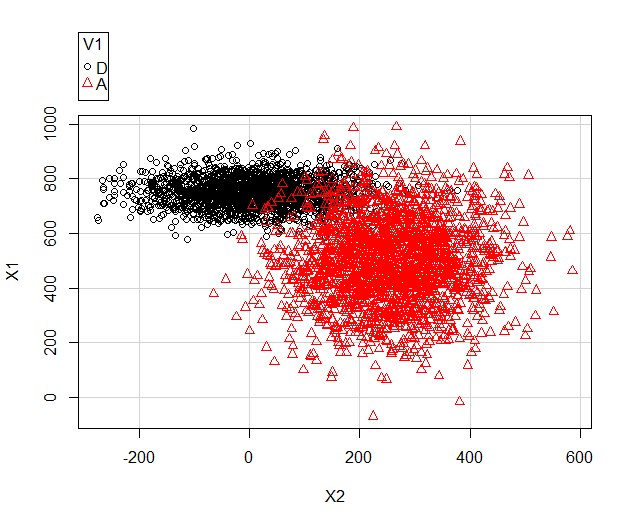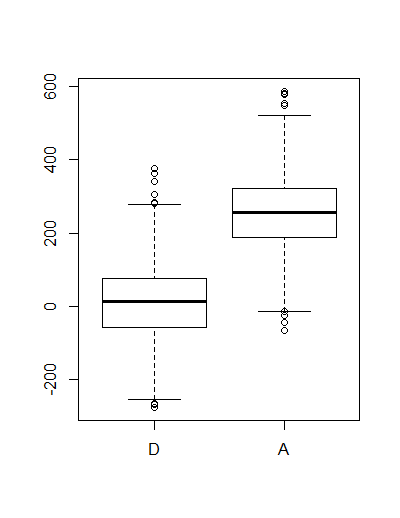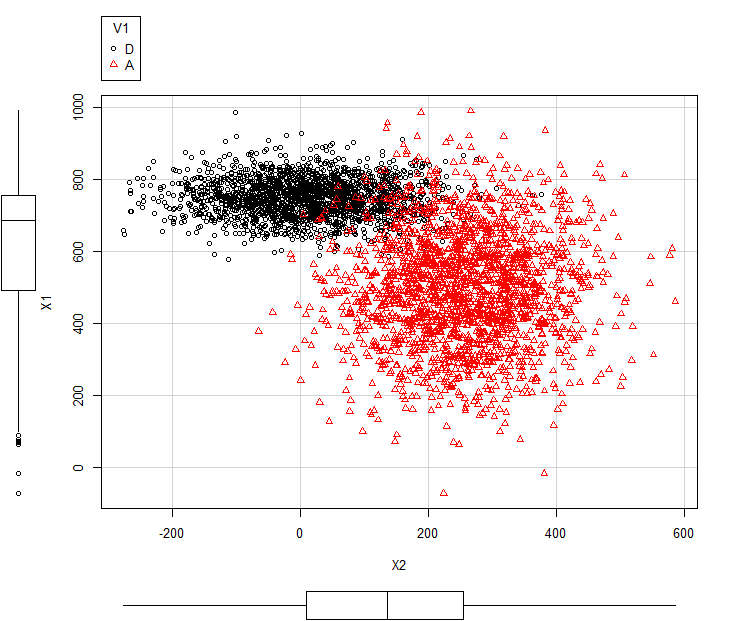# R-Cran Very Basics

`DataFrame<-read.table("clipboard",headers=TRUE)`

Write out the data in a Dataframe into the clipboard:

`write.table(DataFrame,"clipboard-2048",sep="\t")`

You can use this to paste data into other applications.

Create a DataFrame filled with random data (there may be a shorter way of doing this)

```x1<-rnorm(2000,750,55)
x2 <-rnorm(2000,16,100)
df1 <-data.frame(cbind("D",x1,x2))```

Here we've used "cbind" which means bind some columns
the data.frame bit seems to convert something into a collection of columns into a DataFrame.

```x1<-rnorm(2000,500,155)
x2<-rnorm(2000,250,100)
df2<-data.frame(cbind("A",x1,x2))```

Now join them together...

`df0<-data.frame(rbind(df1,df2))`

Now for some reason we have to convert from factors to numerics...

```df0\$X1<-as.numeric(as.character(df0\$x1))
df0\$X2<-as.numeric(as.character(df0\$x2))```

Notice that we've added two extra columns x1 has been converted into X1 and x2 to X2

`str(df0)`

Finally let's plot them:

`scatterplot(X1 ~X2 | V1 ,data=df0,smooth=FALSE,ellipse=FALSE,lty=0)`

Which will look something like this:We can also generate a boxplot using:

```bwplot(X2~V1,data = df0)
boxplot(X2~V1,data = df0)```

bwplot requires the lattice library.Back to the scatter plot graph:

```scatterplot(X1 ~X2 | V1
,data=df0
,smooth=FALSE
,ellipse=FALSE
,lty=0
,grid=TRUE
,boxplots="xy")```

Which gives something like this:Here the boxplots are describing all of X1 and X2  i.e. X1 of  A  and D combined.